Question
1. Write a Python program to print all Perfect numbers between 1 to n. (Use any loop you want)

Perfect number is a positive integer which is equal to the sum of its proper positive divisors.

Proper divisors of 6 are 1, 2, 3.

Sum of its proper divisors = 1 + 2 + 3 = 6.

Hence 6 is a perfect number.

*** proper divisor means the remainder will be 0 when divided by that number.

Sample Input: n = 1000;

Sample Output: 6, 28, 496

```def divisors(n):
s = 1
for x in range(2,n):
if(n%x == 0):
s += x
return s

def isperfect(n):
if(divisors(n) == n):
return True
else:
return False

#Testing
def main():
n = int(input("n = "))
lst = []
for i in range(2,n+1):
if(isperfect(i)):
lst.append(i)
print(str(lst)[1:-1])

main()

```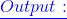```n = 1000
6, 28, 496

```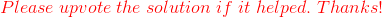#### Earn Coins

Coins can be redeemed for fabulous gifts.

Similar Homework Help Questions
• ### A perfect number is a positive integer that equals the sum of all of its divisors...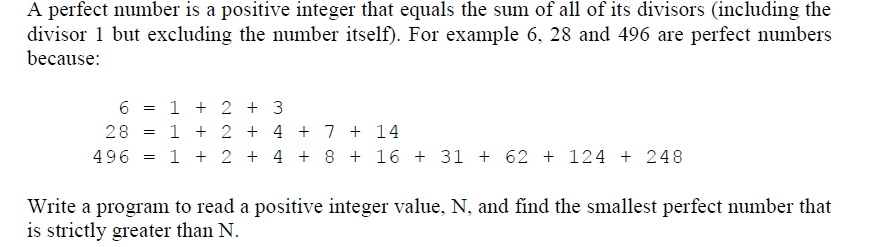A perfect number is a positive integer that equals the sum of all of its divisors (including the divisor 1 but excluding the number itself). For example 6, 28 and 496 are perfect numbers because 6=1+2+3 28 1 + 2 + 4 + 7 + 14 496 1 + 2 + 4 + 8 + 16 + 31 + 62 + 124 + 248 Write a program to read a positive integer value, N, and find the smallest perfect number...

• ### A positive integer is said to be a perfect number if it equals the sum of...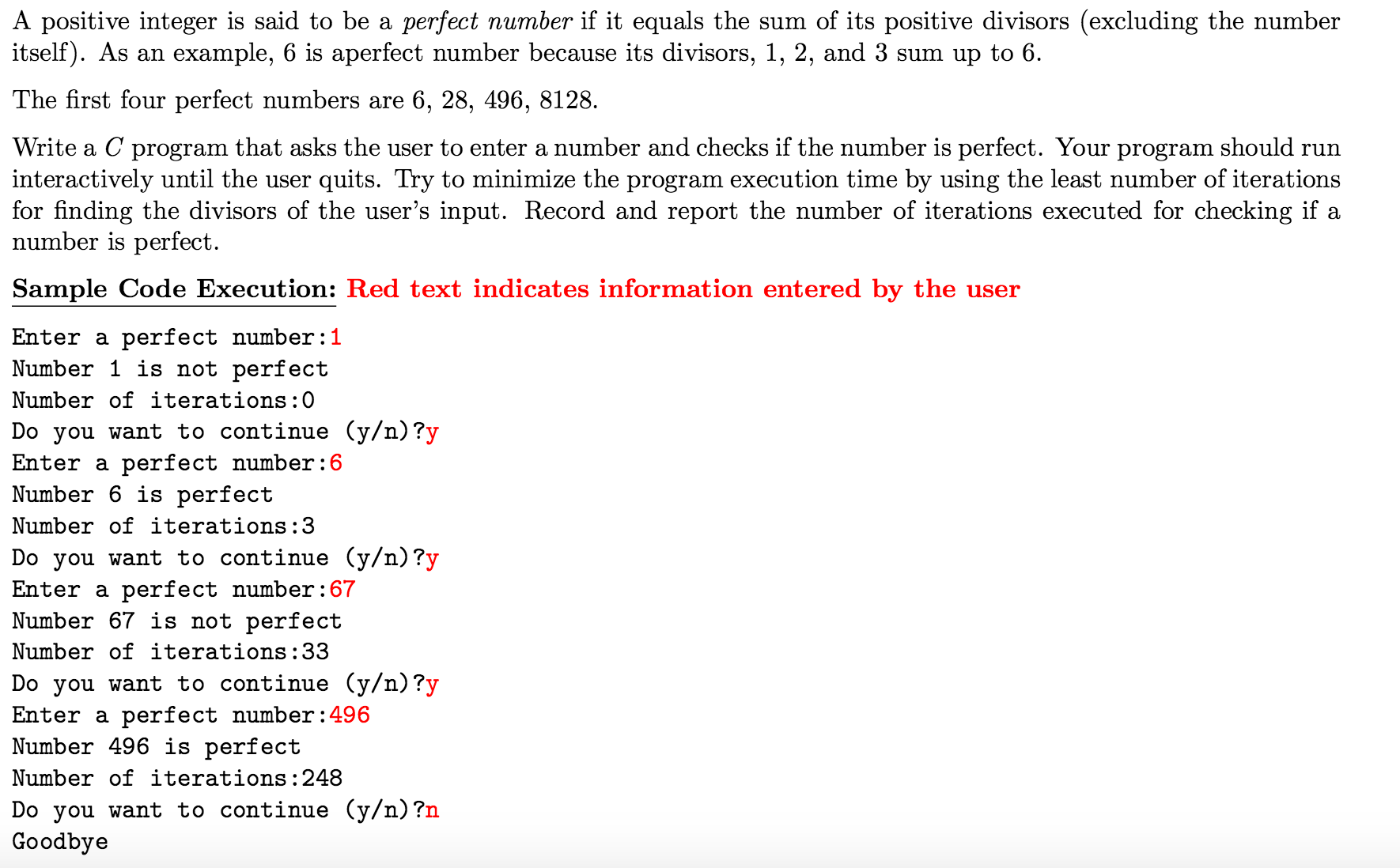A positive integer is said to be a perfect number if it equals the sum of its positive divisors (excluding the number itself). As an example, 6 is aperfect number because its divisors, 1, 2, and 3 sum up to 6. The first four perfect numbers are 6, 28, 496, 8128. Write a C program that asks the user to enter a number and checks if the number is perfect. Your program should run interactively until the user quits. Try...

• ### Using the following functions, modify the Python program written for the perfect number into TDD Java...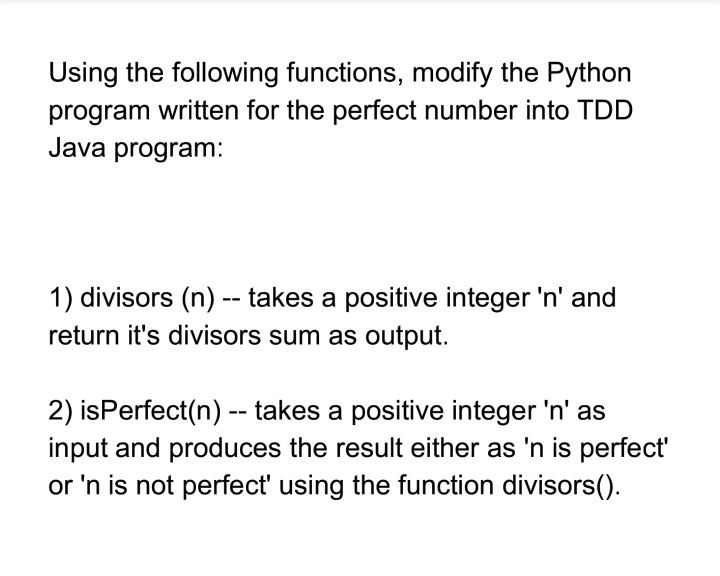Using the following functions, modify the Python program written for the perfect number into TDD Java program: 1) divisors (n) - takes a positive integer 'n' and return it's divisors sum as output. 2) isPerfect(n) - takes a positive integer 'n' as input and produces the result either as 'n is perfect' or 'n is not perfect using the function divisors()

• ### Need help programing this in C. rinteivsors Print the proper divisors of an integer value The...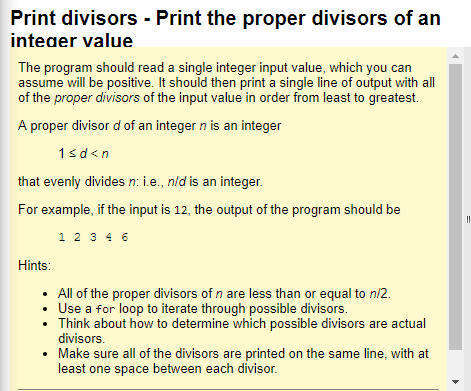Need help programing this in C. rinteivsors Print the proper divisors of an integer value The program should read a single integer input value, which you can assume will be positive. It should then print a single line of output with all of the proper divisors of the input value in order from least to greatest. A proper divisor d of an integer n is an integer that evenly divides n: i.e., nld is an integer For example, if the...

• ### Please Write the task in c++ Task A perfect number is an integer that is equal...

Please Write the task in c++ Task A perfect number is an integer that is equal to the sum of its divisors (where 1 is considered a divisor). For example, 6 is perfect because its divisors are 1, 2, and 3, and 1 + 2 + 3 is 6. Similarly, 28 is perfect because it equals 1 + 2 + 4 + 7 + 14. A quite good number is an integer whose badness—the size of the difference between the...

• ### For C++: A perfect number has these properties 1) positive integer 2) the sum of its...

For C++: A perfect number has these properties 1) positive integer 2) the sum of its proper divisors is the number itself 6 and 28 are perfect numbers Write two functions that return true if its value parameter is a perfect number and false if its value parameter is not a perfect numbers. The two function look like bool isPerfect(int) and void isPerfect(int, bool&) If you want more perfect number you find them on the Internet.

• ### Write a program that asks the user for a lower limit and an upper limit. The...

Write a program that asks the user for a lower limit and an upper limit. The program finds all pairs of amicable numbers where the first number of the pair is between those limits. The second number of the pair may or may not be between the limits. To do this sensibly, write a function int sumDivisors( int num ); that returns the sum of all the proper divisors of num. The main program looks at each integer N between...

• ### Write a Python program that asks users to input an integer number and print the summation...

Write a Python program that asks users to input an integer number and print the summation of its digits and total number of its digits. (i.e., for n=35, sum is 3+5=8, and total number of digits is 2).

• ### Please paste your code and a screenshot of your output! 1. An integer n is divisible...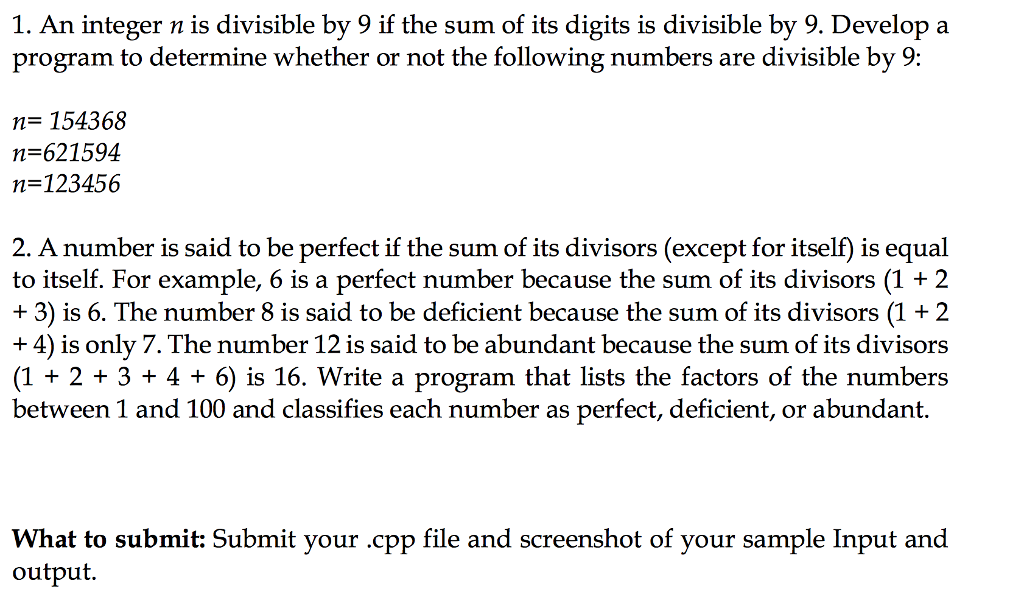Please paste your code and a screenshot of your output! 1. An integer n is divisible by 9 if the sum of its digits is divisible by 9. Develop a program to determine whether or not the following numbers are divisible by 9: n= 154368 n 621594 n-123456 2. A number is said to be perfect if the sum of its divisors (except for itself) is equal to itself. For example, 6 is a perfect number because the sum of...

• ### Lab Goal :   This lab was designed to teach you how to use while and do...

Lab Goal :   This lab was designed to teach you how to use while and do while loops. Lab Description :   A perfect number is any number that is equal to the sum of its divisors. 6 is perfect because it has divisors 1, 2, and 3 which sum to 6.   Determine if the provided numbers are perfect. Use mod (%) to find divisors. Files Needed :: Perfect.java PerfectRunner.java Sample Data : 496 45 6 14 8128 1245 33 28...